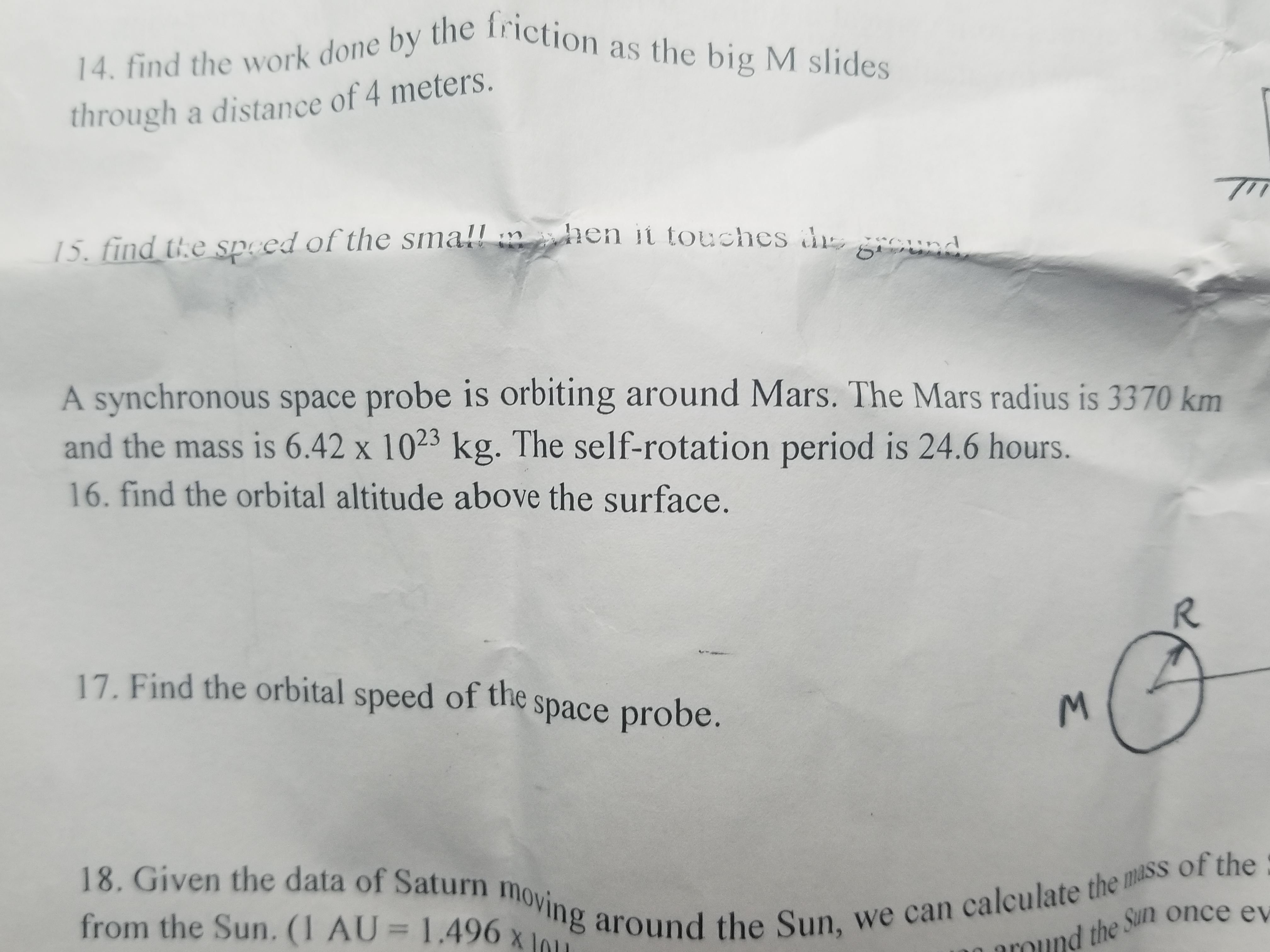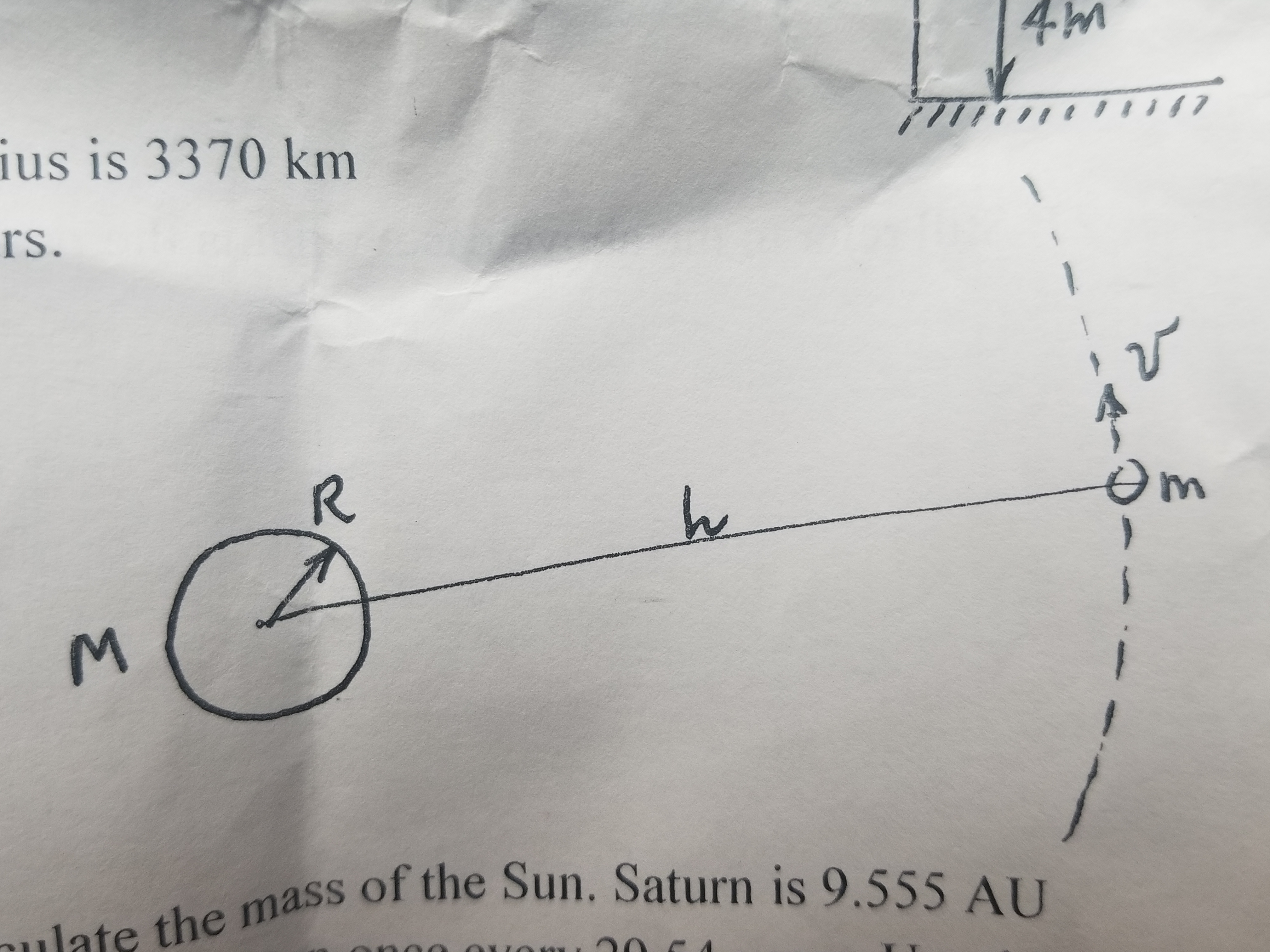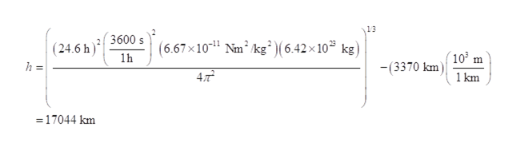# 14. find the work done by the friction as the big M slidesthrough a distance of 4 meters15. find the speed of the smallhen it touches dgOundA synchronous space probe is orbiting around Mars. The Mars radius is 3370 kmand the mass is 6.42 x 1023 kg. The self-rotation period is 24.6 hours.16. find the orbital altitude above the surface.R17. Find the orbital speed of thespace probe.of the18. Given the data of Saturn moving around the Sun, we can calculate the massfrom the Sun. (1 AU = 1.496 xthe Sun once ev 4mllttot t llif7ius is 3370 kmrs.mthe mass of the Sun. Saturn is 9.555 AU

Question
6 views

Question 16

17help_outlineImage Transcriptionclose14. find the work done by the friction as the big M slides through a distance of 4 meters 15. find the speed of the small hen it touches d gOund A synchronous space probe is orbiting around Mars. The Mars radius is 3370 km and the mass is 6.42 x 1023 kg. The self-rotation period is 24.6 hours. 16. find the orbital altitude above the surface. R 17. Find the orbital speed of the space probe. of the 18. Given the data of Saturn moving around the Sun, we can calculate the mass from the Sun. (1 AU = 1.496 x the Sun once ev fullscreenhelp_outlineImage Transcriptionclose4m llttot t llif7 ius is 3370 km rs. m the mass of the Sun. Saturn is 9.555 AU fullscreen
check_circle

Step 1
1. Write an expression for time period using Kepler’s third law
Step 2

Substitute the valueshelp_outlineImage Transcriptionclose13 3600s (6.67x10 Nm2 kg 6.42x 10 kg) (24.6 h) 103 m 1h -(3370 km 4T 1 km = 17044 km fullscreen
Step 3

(17)
Write the expression fo...

### Want to see the full answer?

See Solution

#### Want to see this answer and more?

Solutions are written by subject experts who are available 24/7. Questions are typically answered within 1 hour.*

See Solution
*Response times may vary by subject and question.
Tagged in

### Gravitation